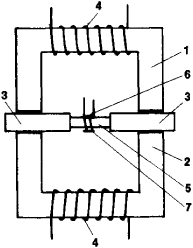Permeameter

Also found in: Dictionary, Wikipedia.

permeameter

[‚pər·mē′äm·əd·ər]
(engineering)
A laboratory device for measurement of permeability of materials, for example, soil or rocks; consists of a powder bed of known dimension and degree of packing through which the particles are forced; pressure drop and rate of flow are related to particle size, and pressure drop is related to surface area.
A device for measuring the coefficient of permeability by measuring the flow of fluid through a sample across which there is a pressure drop produced by gravity.
An instrument for measuring the magnetic flux or flux density produced in a test specimen of ferromagnetic material by a given magnetic intensity, to permit computation of the magnetic permeability of the material.

Permeameter

a device for measuring the magnetic characteristics—usually the magnetization curve and the hysteresis loop—of ferromagnetic specimens having a simple form, such as straight rods, strips, or tubes. It consists of a frame or yoke made of a magnetically soft material and is generally provided with movable parts or pole pieces, which clamp a test specimen in such a way that the specimen forms a closed magnetic circuit with the yoke—it is simpler to determine the magnetic characteristics of a specimen in a closed circuit. A place is provided on the yoke for magnetizing coils and devices for measuring the magnetic induction B and the magnetic field strength H in the specimen.Figure 1. Schematic of a per-meameter: (1) and (2) two halves of yoke, (3) movable pole pieces, (4) magnetizing coils, (5) specimen, (6) winding on specimen for induction measurements, (7) magnetic potentiometer for measuring the strength of the magnetizing field

One type of permeameter whose operation is based on the ballistic method of measurement is shown schematically in Figure 1. The induction B in a specimen is determined by means of a measuring winding connected to the ballistic unit. The strength H is measured by means of a magnetic potentiometer that is connected to the same unit. Field strength can be measured by other means as well, such as Hall transducers, ferromagnetic probes, and magnetic bridges. Other means for determining induction include the use of Hall transducers at the end of the specimen and electrodynamic methods. The magnetic permeability μ of the specimen material is found from the ratio μ = B/H.

REFERENCES

Kifer, 1.1. Ispytaniia ferromagnitnykh materialov, 3rd ed. Moscow, 1969.
Chechernikov, V. I. Magnitnye izmereniia, 2nd ed. Moscow, 1969.
Bozorth, R. Ferromagnetizm. Moscow, 1956. (Translated from English.)

I. I. KIFER

permeameter

An apparatus which measures the permeability, 2 of soils and other similar materials.
Mentioned in ?
References in periodicals archive ?
Finally, the merit of this approach is assessed by comparison of the saturated conductivities obtained via the gas flow technique with values obtained using a conventional permeameter.
The geometric mean of the hydraulic conductivity of 4 years derived from disc permeameter observations at Beverley, Woodanilling, and Cranbrook are presented in Fig.
Infiltration was also measured using modified disc permeameters (Perroux and White 1988), with supply potentials of -4, -3, -2, and -1 cm [H.
The latter reason appears most likely, as this study measured most infiltrations on the plant row and used the disc permeameter to estimate hydraulic conductivities.
S] was taken up again from the data obtained in the laboratory with the permeameter under constant hydraulic head method and the parameter [[psi].
1]) measured in constant head permeameter (LIB ARDI, 2012); and m is obtained from the equation (1).
The permeability of set cement was measured by OFITE Model 360 Air Permeameter (OFI, TEXAS, USA), which could be calculated by the formula shown as follow [18, 19]:
0]) was measured in the leaching columns using the method of constant-load permeameter (EMBRAPA, 1997).
Soil saturated hydraulic conductivity was measured by Guelph Permeameter (Model 2800 KI), taking three steady-state readings.
In accordance with JIS A 1218, hydraulic conductivity was determined using a permeameter (TST-55, Jingkeyusheng, China) .

Site: Follow: Share:
Open / Close